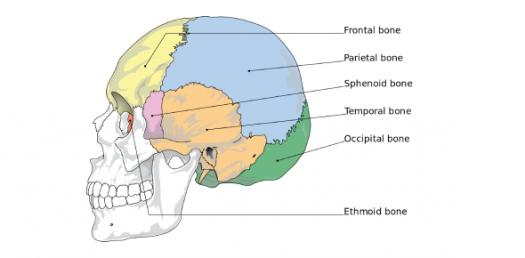# Sphenoid Bone

12 Questions | Total Attempts: 872Settings.

• 1.
What number is 1
• 2.
What number is 2
• 3.
What number is 3 (canal)
• 4.
What number is 4
• 5.
What number is 5 (foramen)
• 6.
What number is 6 (foramen)
• 7.
What number is 7.1 lol
• 8.
What number is 8 (fissure)
• 9.
What number is 9 (foramen)
• 10.
What number is 10
• 11.
What number is 11 (plate)
• 12.
What number is 12
Related Topics